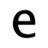remapping values on a chartI wanted to re-map sensor values on a chart. I can do it by using a formula, then charting that value. But it would be nice if this type of option was built into the chart.

There is a good overview of the method I used here here:

http://james-ramsden.com/map-a-value-from-one-number-scale-to-another-formula-and-c-code/

I used formulas to create the mapped values I needed. This is what the formula for the mapped conductivity value is:

(conductivity - min(Sensor.Data.conductivity)) / (max(Sensor.Data.conductivity) - min(Sensor.Data.conductivity))